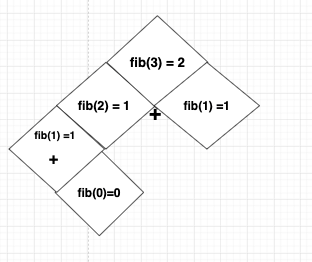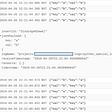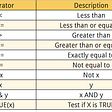# Adding Fibonacci Numbers With Using Recursion Function

`144/89, 1597/987You get the same number:1.618`

# The Problem:

The Fibonacci numbers, commonly denoted `F(n)` form a sequence, called the Fibonacci sequence, such that each number is the sum of the two preceding ones, starting from `0` and `1`. That is,

`F(0) = 0, F(1) = 1F(n) = F(n - 1) + F(n - 2), for n > 1.`
`Input: n = 2Output: 1Explanation: F(2) = F(1) + F(0) = 1 + 0 = 1.`
`Input: n = 3Output: 2Explanation: F(3) = F(2) + F(1) = 1 + 1 = 2.`
`Input: n = 4Output: 3Explanation: F(4) = F(3) + F(2) = 2 + 1 = 3.`

# My Solution

Actually we have a great tip from the question which is mentioning we should use recursion to solve this problem. If you look up the problem description you will see the part which includes as following.

`F(0) = 0, F(1) = 1`
`var fib = function(n) {     if(n === 0 || n ===1){       return n;     }`
`F(n) = F(n - 1) + F(n - 2), for n > 1.`

## We just need to return

`return fib(n-1) + fib(n-2);`
`     //fib = 0,1,2,3,4,5....,N     //Ans = 0,1,1,2,3,5....,?   var fib = function(n) {     if(n === 0 || n ===1){       return n;     }          return fib(n-1) + fib(n-2);}`

## Let’s take a closer look for deep understanding

Example 2:

`Input: n = 2Output: 1Explanation: F(2) = F(1) + F(0) = 1 + 0 = 1.`

## First step

fib(2) = fib(1) + fib(0) which means how we calculate to get the number fib(2) based on Fibonacci Sequence.

` fib = 0,1,2,3,4,5....,N Ans = 0,1,1,2,3,5....,?`
`fib = 0,1,2,3,4,5....,N.   (2)=  0+1,1,2,3,5....,?   fib(1) + fib(0)`
` var fib = function(n) {     if(n === 0 || n ===1){       return n;     }              `Input: n = 3Output: 2Explanation: F(3) = F(2) + F(1) = 1 + 1 = 2.
`fib = 0,1,2,3,4,5....,N   (2)=  0,1+1,2,3,5....,?   fib(1) + fib(2)`
`return fib(n-1) + fib(n-2);    //  1       +  1       = 2`

## Time and Space Complexity

`class Solution {// fib = 0, 1, 2, 3, 4, 5, ..., N// ans = 0, 1, 1, 2, 3, 5, ..., ?// fib(2) = fib(2-1) + fib(2-2)// fib(2) = fib(1) + fib(0)//        =   1 + 0 = 1/*fib(4) = fib(3) + fib(2)/          \fib(3)=2 done     fib(2)/   \            2 is in cache!fib(2)=1 + fib(1)=1/    \fib(1)=1 + fib(0)=0time: O(N), n=4 -> 2^4 = 16space: O(N)*/Map<Integer, Integer> cache = new HashMap<>();// key: N, value: fib(N) answerpublic int fib(int n) {// base case: we already know the answer for nif (n == 0 || n == 1)return n;// base case: already solved answer for nif (cache.containsKey(n))return cache.get(n);// we need to calculate the answerint answer = fib(n - 1) + fib(n - 2);// store this answer for ncache.put(n, answer);return answer;}}`

--

--

--

## More from Soner Mezgitci

Software Engineer | Ruby on Rails | JavaScript | HTML5 | CSS | PostgreSQL | React | Redux

Love podcasts or audiobooks? Learn on the go with our new app.

## How lazy smartapp team uses Slack to stay productive## “If for each object o1 of type S there is an object o2 of type T such that for all programs P…## Python and Stackdriver Logging## Arithmetic & Logical Operators: R Data Types with Example## How to live stream an event from the cloud using Microsoft Teams NDI and OBS.## HackMyVM-Pwned## The Best Product Manager Tools## Soner Mezgitci

Software Engineer | Ruby on Rails | JavaScript | HTML5 | CSS | PostgreSQL | React | Redux

## New TouchOSC Tutorial #4: Receive Data from TouchDesigner with OSC (CHOP)## CS371p Spring 2022: Week 6## Python API Tutorial: Getting Started with APIs## Use Packer from HashiCorp to create images.